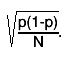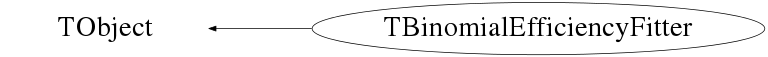# class TBinomialEfficiencyFitter: public TObject

```
TBinomialEfficiencyFitter

Binomial fitter for the division of two histograms.
Use when you need to calculate a selection's efficiency from two histograms,
one containing all entries, and one containing the subset of these entries
that pass the selection, and when you have a parametrization available for
the efficiency as a function of the variable(s) under consideration.

A very common problem when estimating efficiencies is that of error estimation:
when no other information is available than the total number of events N and
the selected number n, the best estimate for the selection efficiency p is n/N.
Standard binomial statistics dictates that the uncertainty (this presupposes
sufficiently high statistics that an approximation by a normal distribution is
reasonable) on p, given N, is$#sqrt{#frac{p(1-p)}{N}}.$
However, when p is estimated as n/N, fluctuations from the true p to its
estimate become important, especially for low numbers of events, and giving
rise to biased results.

When fitting a parametrized efficiency, these problems can largely be overcome,
as a hypothesized true efficiency is available by construction. Even so, simply
using the corresponding uncertainty still presupposes that Gaussian errors
yields a reasonable approximation. When using, instead of binned efficiency
histograms, the original numerator and denominator histograms, a binned maximum
likelihood can be constructed as the product of bin-by-bin binomial probabilities
to select n out of N events. Assuming that a correct parametrization of the
efficiency is provided, this construction in general yields less biased results
(and is much less sensitive to binning details).

A generic use of this method is given below (note that the method works for 2D
and 3D histograms as well):

{
TH1* denominator;              // denominator histogram
TH1* numerator;                // corresponding numerator histogram
TF1* eff;                      // efficiency parametrization
....                           // set step sizes and initial parameter
....                           //   values for the fit function
....                           // possibly also set ranges, see TF1::SetRange()
TBinomialEfficiencyFitter* f = new TBinomialEfficiencyFitter(
numerator, denominator);
Int_t status = f->Fit(eff, "I");
if (status == 0) {
// if the fit was successful, display bin-by-bin efficiencies
// as well as the result of the fit
numerator->Sumw2();
TH1* hEff = dynamic_cast<TH1*>(numerator->Clone("heff"));
hEff->Divide(hEff, denominator, 1.0, 1.0, "B");
hEff->Draw("E");
eff->Draw("same");
}
}

Note that this method cannot be expected to yield reliable results when using
weighted histograms (because the likelihood computation will be incorrect).

```

## Function Members (Methods)

public:
 virtual ~TBinomialEfficiencyFitter() void TObject::AbstractMethod(const char* method) const virtual void TObject::AppendPad(Option_t* option = "") virtual void TObject::Browse(TBrowser* b) static TClass* Class() virtual const char* TObject::ClassName() const virtual void TObject::Clear(Option_t* = "") virtual TObject* TObject::Clone(const char* newname = "") const virtual Int_t TObject::Compare(const TObject* obj) const virtual void TObject::Copy(TObject& object) const virtual void TObject::Delete(Option_t* option = "")MENU virtual Int_t TObject::DistancetoPrimitive(Int_t px, Int_t py) virtual void TObject::Draw(Option_t* option = "") virtual void TObject::DrawClass() constMENU virtual TObject* TObject::DrawClone(Option_t* option = "") constMENU virtual void TObject::Dump() constMENU virtual void TObject::Error(const char* method, const char* msgfmt) const Double_t EvaluateFCN(const Double_t* par) virtual void TObject::Execute(const char* method, const char* params, Int_t* error = 0) virtual void TObject::Execute(TMethod* method, TObjArray* params, Int_t* error = 0) virtual void TObject::ExecuteEvent(Int_t event, Int_t px, Int_t py) virtual void TObject::Fatal(const char* method, const char* msgfmt) const virtual TObject* TObject::FindObject(const char* name) const virtual TObject* TObject::FindObject(const TObject* obj) const TFitResultPtr Fit(TF1* f1, Option_t* option = "") virtual Option_t* TObject::GetDrawOption() const static Long_t TObject::GetDtorOnly() ROOT::Fit::Fitter* GetFitter() virtual const char* TObject::GetIconName() const virtual const char* TObject::GetName() const virtual char* TObject::GetObjectInfo(Int_t px, Int_t py) const static Bool_t TObject::GetObjectStat() virtual Option_t* TObject::GetOption() const virtual const char* TObject::GetTitle() const virtual UInt_t TObject::GetUniqueID() const virtual Bool_t TObject::HandleTimer(TTimer* timer) virtual ULong_t TObject::Hash() const virtual void TObject::Info(const char* method, const char* msgfmt) const virtual Bool_t TObject::InheritsFrom(const char* classname) const virtual Bool_t TObject::InheritsFrom(const TClass* cl) const virtual void TObject::Inspect() constMENU void TObject::InvertBit(UInt_t f) virtual TClass* IsA() const virtual Bool_t TObject::IsEqual(const TObject* obj) const virtual Bool_t TObject::IsFolder() const Bool_t TObject::IsOnHeap() const virtual Bool_t TObject::IsSortable() const Bool_t TObject::IsZombie() const virtual void TObject::ls(Option_t* option = "") const void TObject::MayNotUse(const char* method) const virtual Bool_t TObject::Notify() void TObject::Obsolete(const char* method, const char* asOfVers, const char* removedFromVers) const void TObject::operator delete(void* ptr) void TObject::operator delete(void* ptr, void* vp) void TObject::operator delete[](void* ptr) void TObject::operator delete[](void* ptr, void* vp) void* TObject::operator new(size_t sz) void* TObject::operator new(size_t sz, void* vp) void* TObject::operator new[](size_t sz) void* TObject::operator new[](size_t sz, void* vp) TBinomialEfficiencyFitter& operator=(const TBinomialEfficiencyFitter&) virtual void TObject::Paint(Option_t* option = "") virtual void TObject::Pop() virtual void TObject::Print(Option_t* option = "") const virtual Int_t TObject::Read(const char* name) virtual void TObject::RecursiveRemove(TObject* obj) void TObject::ResetBit(UInt_t f) virtual void TObject::SaveAs(const char* filename = "", Option_t* option = "") constMENU virtual void TObject::SavePrimitive(ostream& out, Option_t* option = "") void Set(const TH1* numerator, const TH1* denominator) void TObject::SetBit(UInt_t f) void TObject::SetBit(UInt_t f, Bool_t set) virtual void TObject::SetDrawOption(Option_t* option = "")MENU static void TObject::SetDtorOnly(void* obj) static void TObject::SetObjectStat(Bool_t stat) void SetPrecision(Double_t epsilon) virtual void TObject::SetUniqueID(UInt_t uid) virtual void ShowMembers(TMemberInspector& insp) const virtual void Streamer(TBuffer&) void StreamerNVirtual(TBuffer& ClassDef_StreamerNVirtual_b) virtual void TObject::SysError(const char* method, const char* msgfmt) const TBinomialEfficiencyFitter() TBinomialEfficiencyFitter(const TBinomialEfficiencyFitter&) TBinomialEfficiencyFitter(const TH1* numerator, const TH1* denominator) Bool_t TObject::TestBit(UInt_t f) const Int_t TObject::TestBits(UInt_t f) const virtual void TObject::UseCurrentStyle() virtual void TObject::Warning(const char* method, const char* msgfmt) const virtual Int_t TObject::Write(const char* name = 0, Int_t option = 0, Int_t bufsize = 0) virtual Int_t TObject::Write(const char* name = 0, Int_t option = 0, Int_t bufsize = 0) const
protected:
 virtual void TObject::DoError(int level, const char* location, const char* fmt, va_list va) const void TObject::MakeZombie()
private:
 void ComputeFCN(Double_t& f, const Double_t* par)

## Data Members

public:
 static TObject::(anonymous) TObject::kBitMask static TObject::EStatusBits TObject::kCanDelete static TObject::EStatusBits TObject::kCannotPick static TObject::EStatusBits TObject::kHasUUID static TObject::EStatusBits TObject::kInvalidObject static TObject::(anonymous) TObject::kIsOnHeap static TObject::EStatusBits TObject::kIsReferenced static TObject::EStatusBits TObject::kMustCleanup static TObject::EStatusBits TObject::kNoContextMenu static TObject::(anonymous) TObject::kNotDeleted static TObject::EStatusBits TObject::kObjInCanvas static TObject::(anonymous) TObject::kOverwrite static TObject::(anonymous) TObject::kSingleKey static TObject::(anonymous) TObject::kWriteDelete static TObject::(anonymous) TObject::kZombie
protected:
 Bool_t fAverage True if the fit function must be averaged over the bin TH1* fDenominator Denominator histogram Double_t fEpsilon Precision required for function integration (option "I") Bool_t fFitDone Set to kTRUE when the fit has been done ROOT::Fit::Fitter* fFitter pointer to the real fitter TF1* fFunction Function to fit TH1* fNumerator Numerator histogram Bool_t fRange True if the fit range must be taken from the function range

## Class Charts## Function documentation

``` default constructor
```
TBinomialEfficiencyFitter(const TH1* numerator, const TH1* denominator)
``` Constructor.

Note that no objects are copied, so it is up to the user to ensure that the
histogram pointers remain valid.

Both histograms need to be "consistent". This is not checked here, but in
TBinomialEfficiencyFitter::Fit().
```

``` destructor
```
void Set(const TH1* numerator, const TH1* denominator)
``` Initialize with a new set of inputs.
```
void SetPrecision(Double_t epsilon)
``` Set the required integration precision, see TF1::Integral()
```
ROOT::Fit::Fitter* GetFitter()
``` Provide access to the underlying fitter object.
This may be useful e.g. for the retrieval of additional information (such
as the output covariance matrix of the fit).
```
TFitResultPtr Fit(TF1* f1, Option_t* option = "")
``` Carry out the fit of the given function to the given histograms.

If option "I" is used, the fit function will be averaged over the
bin (the default is to evaluate it simply at the bin center).

If option "R" is used, the fit range will be taken from the fit
function (the default is to use the entire histogram).

If option "S" a TFitResult object is returned and it can be used to obtain
additional fit information, like covariance or correlation matrix.

Note that all parameter values, limits, and step sizes are copied
from the input fit function f1 (so they should be set before calling
this method. This is particularly relevant for the step sizes, taken
to be the "error" set on input, as a null step size usually fixes the
corresponding parameter. That is protected against, but in such cases
an arbitrary starting step size will be used, and the reliability of
the fit should be questioned). If parameters are to be fixed, this
should be done by specifying non-null parameter limits, with lower
limits larger than upper limits.

On output, f1 contains the fitted parameters and errors, as well as
the number of degrees of freedom, and the goodness-of-fit estimator
as given by S. Baker and R. Cousins, Nucl. Instr. Meth. A221 (1984) 437.
```
void ComputeFCN(Double_t& f, const Double_t* par)
``` Compute the likelihood.
```
Double_t EvaluateFCN(const Double_t* par)